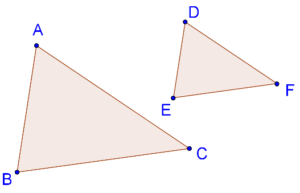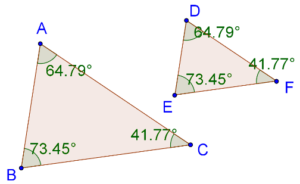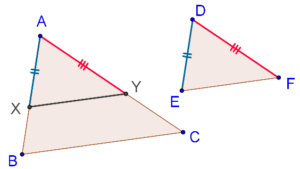# SSS Criterion in Triangles

SSS Criterion in Triangles

## Introduction to similarity:

If two triangles are similar it means that:

• All corresponding angle pairs are equal
• All corresponding sides are proportional

However, in order to be sure that two triangles are similar, we do not necessarily need to have information about all sides and all angles.

## What is SSS similarity criterion?

The SSS similarity criterion states that if the three sides of one triangle are respectively proportional to the three sides of another, then the two triangles are similar.

This essentially means that any such pair of triangles will be equiangular(All corresponding angle pairs are equal) also. Consider the following figure, in which the sides of two triangles ($$\Delta ABC$$ and $$\Delta DEF$$) are respectively proportional:That is, it is given that:

$\frac{{AB}}{{DE}} = \frac{{BC}}{{EF}} = \frac{{AC}}{{DF}}$

Can we say that these two triangles will be equiangular? The SSS criterion says that we can. Indeed, if we measure the angles in each triangle, we will find this to be true, as the following figure shows:## Proof of SSS similarity criterion:

Suppose that $$AB > DE$$. Then $$AC$$ will also be greater than $$DF$$ (why).

Given: $$\frac{{DE}}{{AB}} = \frac{{DF}}{{AC}} = \frac{{EF}}{{BC}}$$

To prove:  $$\Delta DEF$$ is similar to $$\Delta ABC$$

Construction:

1.  Take a point $$X$$ on $$AB$$ such that $$AX = DE$$

2.  Take a point $$Y$$ on $$AC$$ such that $$AY = DF$$

3. Join $$XY$$Proof: It is given that

$\frac{{DE}}{{AB}} = \frac{{DF}}{{AC}}$

Thus,

$\frac{{AX}}{{AB}} = \frac{{AY}}{{AC}}(\because AX = DE,AY = DF)$

Using the converse of the BPT(If a line divides any two sides of a triangle in the same ratio, then the line must be parallel to the third side), this implies that $$XY\parallel BC$$. Thus, $$\Delta AXY$$ ~ $$\Delta ABC$$. This further means that:

$\frac{{AX}}{{AB}} = \frac{{AY}}{{AC}} = \frac{{XY}}{{BC}}$

But we also have:

$\frac{{EF}}{{BC}} = \frac{{AY}}{{AC}} = \frac{{AX}}{{AB}}$

Thus,

\begin{align} \frac{{XY}}{{BC}} &= \frac{{EF}}{{BC}}\\ \Rightarrow XY &= EF \end{align}

Now, by the SSS congruence criterion,

\begin{align} &\Delta DEF \cong \Delta AXY \hfill \\ \Rightarrow &\Delta DEF \sim \Delta AXY....(1) \hfill \\ \end{align}

While we already have, $$\Delta AXY \sim \Delta ABC....(2)$$

Thus from (1) and (2),

$\boxed{\Delta DEF \sim \Delta ABC}$

This concludes our proof.

Challenge: The dimensions of $$\Delta ABC$$ and $$\Delta DEF$$ are as follows:

$$AB$$ = 5 units, $$BC$$ = 4 units, $$AC$$ = 6 units, $$DE$$ = 15 units, $$EF$$ = 6 units, $$DF$$ = 18 units.

Are they similar? If yes, by which criterion?

⚡Tip: Match the longest side with the longest side and the shortest side with the shortest side and check all three ratios.